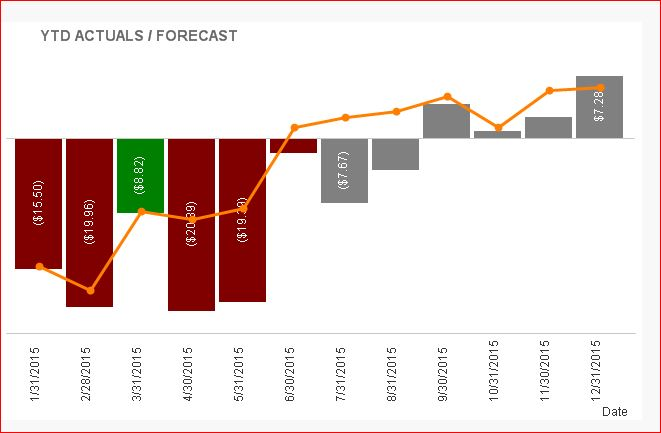# QlikView App Dev

Discussion Board for collaboration related to QlikView App Development.

Announcements
Join this live chat April 6, 10AM EST - QlikView to Qlik Sense REGISTER
cancel
Showing results for
Search instead for
Did you mean:Not applicable

## Conditional Format Date Range

I browsed the community and still am having trouble formatting values based on a given date range. For values that are less than the current month, I want to format them either red or green, while values greater than or equal to the current month colored grey. My current background color expression is as follows, any suggestions?

=
IF(
(
Sum({\$<[Sales Data.Metric]={'Direct Revenue'}, [FiscalMonth]={">=\$(=Max(FiscalMonth)<=\$(=Max(FiscalMonth)"}> [Sales Data.Actual])
-
Sum({\$<[Sales Data.Metric]={'Direct Revenue'}, [FiscalMonth]={">=\$(=Max(FiscalMonth)<=\$(=Max(FiscalMonth)"}>} [Sales Data.Plan])) < 0,
rgb(219,60,36),
IF(
(
Sum({\$<[Sales Data.Metric]={'Direct Revenue'}, [FiscalMonth]={">=\$(=Max(FiscalMonth)<=\$(=Max(FiscalMonth)"}> [Sales Data.Actual])
-
Sum({\$<[Sales Data.Metric]={'Direct Revenue'}, [FiscalMonth]={">=\$(=Max(FiscalMonth)<=\$(=Max(FiscalMonth)"}>} [Sales Data.Plan])) >= 0,
Green(),
rgb(128, 128, 128)))

1 Solution

Accepted SolutionsMVP

Hi Michael,

May be like this.I'm in the learning level. May be some expert will give the good idea.

21 RepliesMVP

Is your FiscalMonth a numeric field? or does it look like Jan, Feb..and so on?Not applicable
Author

Jan, Feb, ...., does it need to be numeric?MVP

Max(FiscalMonth) converts the format of Month into Numeric field. Do you have a numeric equivalent of FiscalMonth in your data??? Something like FiscalMonthNoNot applicable
Author

Yes, DN = The numerical value of the date, ####MVP

See if this helps:

=IF(
(
Sum({\$<[Sales Data.Metric]={'Direct Revenue'}, DN={">=\$(=MonthStart(Date(Max(DN)))) <=\$(=MonthEnd(Date(Max(DN))))"}> [Sales Data.Actual])
-
Sum({\$<[Sales Data.Metric]={'Direct Revenue'}, DN={">=\$(=MonthStart(Date(Max(DN)))) <=\$(=MonthEnd(Date(Max(DN))))"}>} [Sales Data.Plan])) < 0,
rgb(219,60,36),
IF(
(
Sum({\$<[Sales Data.Metric]={'Direct Revenue'}, DN={">=\$(=MonthStart(Date(Max(DN)))) <=\$(=MonthEnd(Date(Max(DN))))"}>} [Sales Data.Actual])
-
Sum({\$<[Sales Data.Metric]={'Direct Revenue'}, DN={">=\$(=MonthStart(Date(Max(DN)))) <=\$(=MonthEnd(Date(Max(DN))))"}>} [Sales Data.Plan])) >= 0,
Green(),
rgb(128, 128, 128)))MVP

This might help:

Why don’t my dates work?

Logic will get you from a to b. Imagination will take you everywhere. - A EinsteinNot applicable
Author

Still not working. I simply want to write out for the first f(x) if month is between MIN(DN) and Current(DN).Master III

{">=\$(=Max(FiscalMonth)<=\$(=Max(FiscalMonth)"}

Do you only want the data for max(FiscalMonth)?.. It looks wrong to meNot applicable
Author

No, for the first and second IF() I want dates between JAN and Today, then for the third and fourth I want dates greater than or equal to Today.